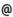## "Babes-Bolyai" University of Cluj-Napoca Faculty of Mathematics and Computer Science

 Geometric function theory
 Code Semes-ter Hours: C+S+L Credits Type Section MMC0002 5 2+1+0 4 optional Matematică MMC0002 5 2+1+0 5 optional Matematici aplicate
 Teaching Staff in Charge
 Prof. BULBOACA Teodor, Ph.D., bulboacamath.ubbcluj.ro
 Acad. MOCANU Petru, Ph.D., pmocanumath.ubbcluj.ro
 Aims The presentation of principal classes of univalent functions defined by remarkable geometric properties and some of their applications in the theory of conformal mappings.
 Content 1. Univalent functions; classical results. Aria's Theorem. Covering Theorem for the S class (Koebe, Bieberbach). Covering Theorem for the Sigma class. Distortion Theorems (Koebe, Bieberbach). The compactness of the S class. The Bieberbach's conjecture. 2. Analytical functions with real positive part. Subordination. - Integral representation; Herglotz's formula. The theorems of Herglotz. - Representations by Stiltjes integrals. Caratheodory's Theorem. - Bounds of holomorphic functions with real positive part. - Subordination; the subordination principle (Lindelof). Sakaguchi's Lemma. 3. Special classes of univalent functions. - Starlike functions. Radius of starlikeness. Theorem about the coefficient bounds of functions from S^*. Structure formula. - Convex functions. Duality's Theorem (Alexander). The compactness of K class. Radius of convexity. - Alpha - convex functions. The Theorem of starlikeness of alpha - convex functions. Duality's Theorem. Radius of alpha - convexity. Bounds Theorems (Miller). - Close - to - convex functions. Univalence criteria of Noshiro - Warschawski - Wolff. Univalence criteria of Ozaki - Kaplan. Characterizing Theorem of close - to - convex functions (Kaplan). Linear accessible domains. - Typical real functions. Structure formula. Duality Theorem. Theorem about the coefficients. A sufficient condition for the univalence of the typical real functions. Consequence (Aksentiev). Thalk - Chakalov Theorem. Univalence criteria for meromorphic functions. Aksentiev's Theorem. Starlikeness and convexity conditions for meromorphic functions. 4. Diffeomorphism conditions in the complex plane. - Spirallike generalized functions of C^1 class. General theorems; particular cases. - Nonanalytic alpha - convex function. Preliminary lemmas. The Theorem of starlikeness of alpha - convex nonanalytic functions. Examples. - C^1 transforms and the refraction law. - Close- to -convex functions of C^1 class. Fundamental theorems. Particular cases. Examples.
 References 1. GOLUZIN, G. M. : Geometric theory of functions of a complex variable, Trans. Math. Mon., Amer. Math. Soc., 1969. 2. GOODMAN, A. W. : Univalent functions (vol. I, II), Mariner Publishing Co., Tampa, 1983. 3. DUREN, P. L. : Univalent functions, Springer Verlag, Berlin, Heidelberg, 1984. 4. MOCANU, PETRU - BULBOACĂ, TEODOR - SĂLĂGEAN, GR. ŞTEFAN : Teoria geometrică a funcţiilor univalente, Casa Cărţii de Ştiinţă, Cluj-Napoca, 1999. 5. BULBOACĂ, TEODOR - MOCANU, PETRU : Bevezetés az analitikus függvények geometriai elméletébe, Editura Abel (Erdely Tankönyvtanács), Cluj-Napoca, 2003. 6. GRAHAM, IAN - KOHR, GABRIELA : Geometric function theory in one and higher dimensions, M. Dekker, 2003.
 Assessment Exam. Student tests during the semester; their average represents 1/3 from the final score.